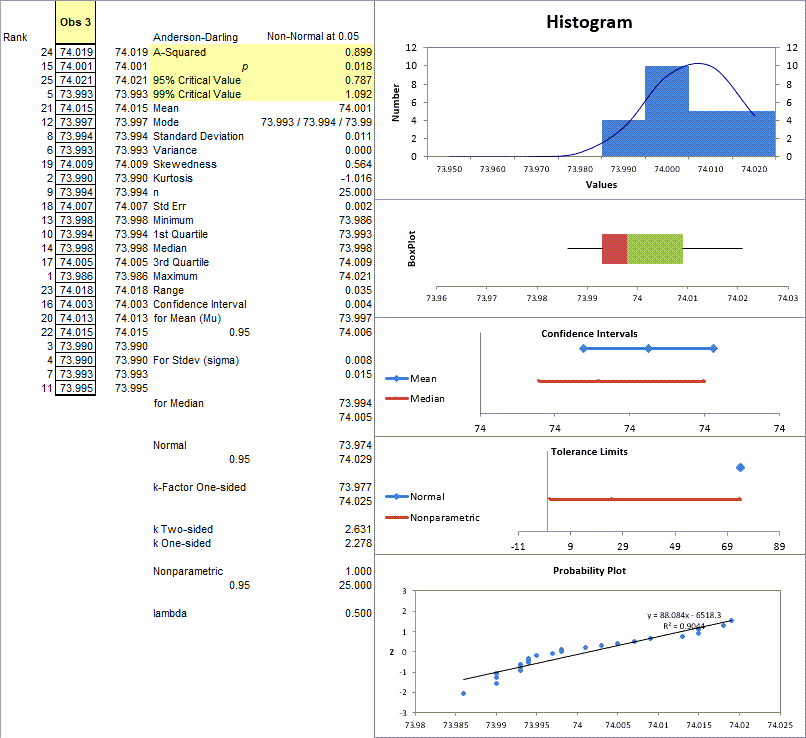# Trying to Do Anderson Darling Normality Tests in Excel?

## QI Macros can do it for you!

### Create an Anderson-Darling Normality Test Using QI Macros:

2. Click on QI Macros menu > Statistical Tools > Descriptive Statistics
3. QI Macros will do the math, draw the charts and analyze results for you.

### Go Deeper: Is Your Data Normal?

Statistical analysis, like ANOVA, may rely on your data being "normal" (i.e., bell-shaped). So how can you tell if your data is normal?

### Anderson Darling p-value and Critical Value Method

QI Macros add-in for Excel contains a Normality Test which uses the Anderson-Darling method. The output includes the Anderson-Darling statistic, A-squared, and both a p-value and critical values for A-squared.

Note: Excel does not do an Anderson Darling test;

QI Macros adds a new tab to Excel's menu. To run a normality test using QI Macros:

1. Just select your data, then click on the QI Macros menu and select Statistical Tools > Descriptive Statistics - Normality Test:2. QI Macros will run an Anderson-Darling Normality Test and other descriptive statistics giving both numerical and graphical representations of the data:### Interpreting the Anderson Darling test results

#### The Anderson-Darling values shown are:

• A-squared = 0.270
• p value= 0.648
• 95% Critical Value = 0.787
• 99% Critical Value = 1.092
 If Then p value <= a Reject the null hypothesis p value > a Cannot reject the null hypothesis (accept null hypothesis) - the data is normal. A-squared > critical value Reject the null hypothesis A-squared <= critical value Cannot reject the null hypothesis - the data is normal.

"Null hypothesis" is that the data is normal.

The "alternative hypothesis" is that the data is non-normal.

Reject the Null hypothesis (i.e., accept the alternative) when p<=alpha or A-squared>critical value.

Using the p value:  p = 0.648 which is greater than alpha (level of significance) of 0.01. So we cannot reject the null hypothesis (accept the null hypothesis) (i.e., the data is normal).

Using the critical values, you would only reject this "null hypothesis" (i.e., data is non-normal) if A-squared is greater than either of the two critical values. Since 0.270 < 0.787 and 0.270 < 1.092, you can be at least 99% confident that the data is normal.

Show less

### Another Example

Using Cells D1:D41 (after deleting the blank row) from the XbarR.xlsx in c:\program files\qimacros\testdata, you would get the following result:Notice how the normality plot curves at the right so that some of the points are farther from the line? Using Anderson-Darling we discover that the data is considered normal at one level (99%), but not at another (95%).

Using the p value p = 0.018 which is greater than alpha of 0.01 (0.01 < 0.018 < 0.05), we can reject the null hypothesis (i.e., the data is normal) at alpha = 0.05, but not at alpha = 0.01.

Using the critical values, . Since 0.787 < 0.899 < 1.092, you would reject the null hypothesis at 95% but not reject it at 99%.

Frankly, the double negatives of "not rejecting the null hypothesis" makes my brain tired. All I really want to know is: "Is my data normal?" So, in summary:

• if the dots fit the trend line on the normal probability plot, then the data is normal.
• if p > alpha then the data is normal.
• if A-squared < Critical Value, then the data is normal.

### Why Choose QI Macros Statistical Software for Excel?### Easy to Use

• Works Right in Excel
• Interprets p-values for You
• Accurate No-Worry Results
• Free Training Anytime### Proven and Trusted

• 100,000 Users in 80 Countries
• Celebrating 20th Anniversary
• Five Star CNET Rating - Virus Free### Affordable

• Only \$349 USD
Quantity Discounts Available
• No annual fees
• Free Technical Support